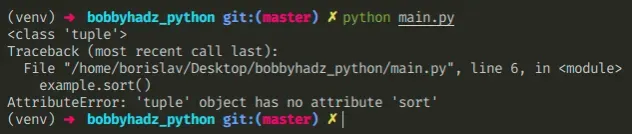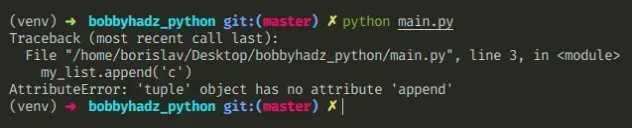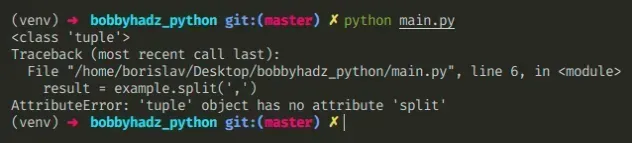# AttributeError: 'tuple' object has no attribute X in Python7 min## #AttributeError: 'tuple' object has no attribute X in Python

The Python "AttributeError: 'tuple' object has no attribute" occurs when we access an attribute that doesn't exist on a tuple.

To solve the error, use a list instead of a tuple to access a list method or correct the assignment.Here is an example of how the error occurs.

main.py
```Copied!```example = 'bobby', 'hadz'

print(type(example))  # 👉️ <class 'tuple'>

# ⛔️ AttributeError: 'tuple' object has no attribute
example.sort()
``````

The `example` variable stores a tuple of 2 values.

main.py
```Copied!```example = 'bobby', 'hadz'

``````
Tuples are very similar to lists, but implement fewer built-in methods and are immutable (cannot be changed).

## #Using a list instead of a tuple

If you meant to access a list method, use a list instead of a tuple.

main.py
```Copied!```example = ['bobby', 'hadz', 'com']

print(type(example))  # 👉️ <class 'list'>

example.sort()

print(example) # 👉️ ['bobby', 'com', 'hadz']
``````

Lists are wrapped in square brackets `[]` and tuples are wrapped in parentheses `()`.

## #Converting a tuple to a list

You can convert a tuple to a list by using the `list()` constructor.

main.py
```Copied!```my_tuple = ('bobby', 'hadz', 'com')

# ✅ convert a tuple to list
my_list = list(my_tuple)

print(my_list)  # 👉️ ['bobby', 'hadz', 'com']
print(type(my_list))  # 👉️ <class 'list'>
``````

The list class takes an iterable and returns a list object.

## #How tuples are constructed in Python

If you created the tuple by mistake, you have to correct the assignment.

Tuples are constructed in multiple ways:

• Using a pair of parentheses `()` creates an empty tuple
• Using a trailing comma - `a,` or `(a,)`
• Separating items with commas - `a, b` or `(a, b)`
• Using the `tuple()` constructor

## #Accessing a tuple element at a specific index

If you meant to access an element at a specific index in a tuple, use square brackets.

main.py
```Copied!```my_tuple = ('bobby', 'hadz', 'com')

print(my_tuple.upper())  # 👉️ "BOBBY"
``````
Python indexes are zero-based, so the first item in a tuple has an index of `0`, and the last item has an index of `-1` or `len(my_tuple) - 1`.

## #Track down where the variable got assigned a tuple

If you created the tuple by mistake, track down where the variable got assigned a tuple and correct the assignment.

Here is an example of creating a tuple by mistake by separating values with a comma.

main.py
```Copied!```def get_list():
return 'a', 'b' # 👈️ returns a tuple

# 👇️ ('a', 'b')  tuple
result = get_list()

# ⛔️ AttributeError: 'tuple' object has no attribute 'append'
result.append('c')
``````

The `get_list` function returns a tuple instead of a list because we didn't wrap the values in square brackets.

To correct the error in this scenario, wrap the items in square brackets.

main.py
```Copied!```
def get_list():
return ['a', 'b']

# 👇️ ['a', 'b']  list
result = get_list()

result.append('c')

print(result)  # 👉️ ['a', 'b', 'c']
``````

Make sure you don't have any dangling commas after a value, e.g. `a,` or `(a, )` because you could be creating a tuple by mistake.

Tuple objects have very few built-in methods and are immutable (cannot be changed), so chances are you meant to create a different type of object.

## #Creating a dictionary instead of a tuple

If you meant to create a dictionary, wrap key-value pairs in curly braces.

main.py
```Copied!```employee = {'name': 'Bobby Hadz', 'age': 30}

print(employee['age']) # 👉️ 30
``````

A dictionary is used to store key-value pairs.

## #There are only 2 methods that you will use on a tuple object

There are only 2 methods that you will likely be using on tuple objects.

main.py
```Copied!```my_tuple = ('a', 'b', 'c', 'c')

print(my_tuple.count('c'))  # 👉️ 2
print(my_tuple.index('a'))  # 👉️ 0
``````

The `count` method returns the number of occurrences of the value in the tuple and the `index` method returns the index of the value in the tuple.

If you need to mutate the sequence, you have to use a `list` because tuples are immutable.

You can view all the attributes an object has by using the `dir()` function.

main.py
```Copied!```my_tuple = ('a', 'b', 'c', 'c')

# 👇️ [... 'count', 'index' ...]
print(dir(my_tuple))
``````

If you pass a class to the dir() function, it returns a list of names of the class's attributes, and recursively of the attributes of its bases.

If you try to access any attribute that is not in this list, you would get the error.

## #Solving the error for specific methods

Here are 2 examples of solving the error for specific methods. Click on the link to navigate to the subheading.

## #AttributeError: 'tuple' object has no attribute 'append'

The Python "AttributeError: 'tuple' object has no attribute 'append'" occurs when we try to call the `append()` method on a tuple instead of a list.

To solve the error, use a list instead of a tuple because tuples are immutable.Here is an example of how the error occurs.

main.py
```Copied!```my_list = ('a', 'b')

print(type(my_list))  # 👉️ <class 'tuple'>

# ⛔️ AttributeError: 'tuple' object has no attribute 'append'
my_list.append('c')
``````

We used parentheses to wrap the comma-separated elements, so we ended up creating a tuple object.

Tuples are very similar to lists, but implement fewer built-in methods and are immutable (cannot be changed).

## #Use a list instead of a tuple

To solve the error, we have to use a list instead of a tuple.

main.py
```Copied!```my_list = ['a', 'b']

print(type(my_list))  # 👉️ <class 'list'>

my_list.append('c')

print(my_list)  # 👉️ ['a', 'b', 'c']
``````

We wrapped the items in square brackets to create a list and we were able to call the `append()` method to add an item to the list.

Note that to create an empty list, you would use square brackets, e.g. `my_list = []` and not parentheses.

## #Converting a tuple to a list

You can convert a tuple into a list by using the `list()` constructor.

main.py
```Copied!```my_tuple = ('a', 'b')

my_list = list(my_tuple)

my_list.append('c')

print(my_list)  # 👉️ ['a', 'b', 'c']
``````
Tuple objects implement very few methods because they are immutable (cannot be changed). This is the reason tuple objects don't implement methods like `append()` which change the object in place.

Note that the `append()` method mutates the original list, it doesn't return a new list.

The `append()` method returns None, so don't assign the result of calling it to a variable.

If you need to mutate the sequence, you have to use a `list` because tuples are immutable.

There are only 2 methods that you will likely be using on tuple objects.

main.py
```Copied!```my_tuple = ('a', 'b', 'c', 'c')

print(my_tuple.count('c'))  # 👉️ 2
print(my_tuple.index('a'))  # 👉️ 0
``````

The `count` method returns the number of occurrences of the value in the tuple and the `index` method returns the index of the value in the tuple.

You can view all the attributes an object has by using the `dir()` function.

main.py
```Copied!```my_tuple = ('a', 'b', 'c', 'c')

# 👇️ [... 'count', 'index' ...]
print(dir(my_tuple))
``````

If you pass a class to the dir() function, it returns a list of names of the class's attributes, and recursively of the attributes of its bases.

If you try to access any attribute that is not in this list, you would get the error.

## #AttributeError: 'tuple' object has no attribute 'split'

The Python "AttributeError 'tuple' object has no attribute 'split'" occurs when we call the `split()` method on a tuple instead of a string.

To solve the error, correct the assignment of the variable or access the tuple at a specific index when calling `split()`.## #Make sure you haven't declared a tuple by mistake

Here is an example of how the error occurs.

main.py
```Copied!```example = 'bobby,hadz', # 👈️ dangling comma declares tuple

print(type(example))  # 👉️ <class 'tuple'>

# ⛔️ AttributeError: 'tuple' object has no attribute 'split'
result = example.split(',')
``````

We declared a tuple instead of a string by separating multiple values with a comma.

Tuples are very similar to lists, but implement fewer built-in methods and are immutable (cannot be changed).

To be able to use the `split()` method, we have to declare a string or access the tuple at a specific index.

main.py
```Copied!```example = 'bobby,hadz'

print(type(example))  # 👉️ <class 'string'>

# ⛔️ AttributeError: 'tuple' object has no attribute 'split'
result = example.split(',')

``````

We declared a string, so we were able to use the `split()` method to split the string on each comma.

## #Access a specific tuple element before calling split()

If you meant to call `split()` on an element of a tuple, access it at the specific index.

main.py
```Copied!```my_tuple = ('a,b', 'c,d')

result = my_tuple.split(',')

print(result) # 👉️ ['c', 'd']
``````

We accessed the tuple element at index `1` which is a string, so we were able to call the `split()` method.

Python indexes are zero-based, so the first item in a tuple has an index of `0`, and the last item has an index of `-1` or `len(my_tuple) - 1`.

## #How tuples are constructed

Tuples are constructed in multiple ways:

• Using a pair of parentheses `()` creates an empty tuple
• Using a trailing comma - `a,` or `(a,)`
• Separating items with commas - `a, b` or `(a, b)`
• Using the `tuple()` constructor

Note that tuples are immutable, so if you have to mutate a sequence, you have to use a `list` instead.

There are only 2 methods that you will likely be using on tuple objects.

main.py
```Copied!```my_tuple = ('a', 'b', 'c', 'c')

print(my_tuple.count('c'))  # 👉️ 2
print(my_tuple.index('a'))  # 👉️ 0
``````

The `count` method returns the number of occurrences of the value in the tuple and the `index` method returns the index of the value in the tuple.

You can view all the attributes an object has by using the `dir()` function.

main.py
```Copied!```my_tuple = ('bobby', 'hadz', 'com')

# 👇️ [... 'count', 'index' ...]
print(dir(my_tuple))
``````

If you pass a class to the dir() function, it returns a list of names of the class's attributes, and recursively of the attributes of its bases.

If you try to access any attribute that is not in this list, you would get the "AttributeError: tuple object has no attribute" error.